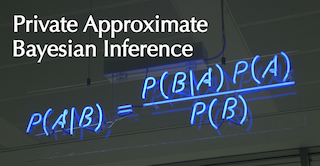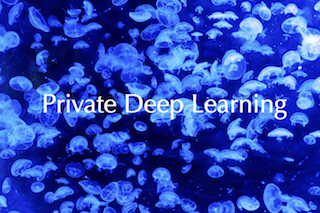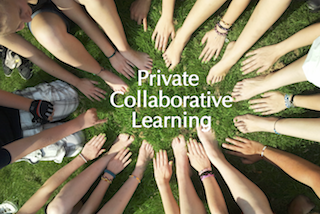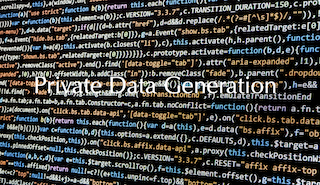Our research group focuses on developing practical algorithms for privacy preserving machine learning.

We are particularly interested in the following research themes, among many others :(0) How can we privatize widely used approximate Bayesian inference methods? As an initial attempt, we made the stochastic variational inference differentially private, applied to topic modeling, Bayesian logistic regression, and Sigmoid belief networks.(1) The bigger the model gets, the better the model memorizes the data. How can we privatize the model parameters in big models like deep neural networks (DNNs) to avoid revealing sensitive information on the training data? What is the most effective way to privatize tens to hundreds of millions of model parameters in DNNs given a limited privacy budget?(2) In collaborative (federated, or distributed) learning, where data is distributed among many data owners who are willing to collaborate to develop statistical tools together (e.g., many hospitals aim to develop a classifier to detect a certain disease) , what would be the most efficient way to share their locally learned models with others without leaking sensitive information about their data?(3) Can we learn a data distribution from a dataset in a "private" way, such that the learned distribution preserves privacy? If so, then we can generate data samples from the learned distribution, and release as many samples as one needs for further statistical analyses without worrying about privacy.

news
I have become a CIFAR AI chair. The news is here